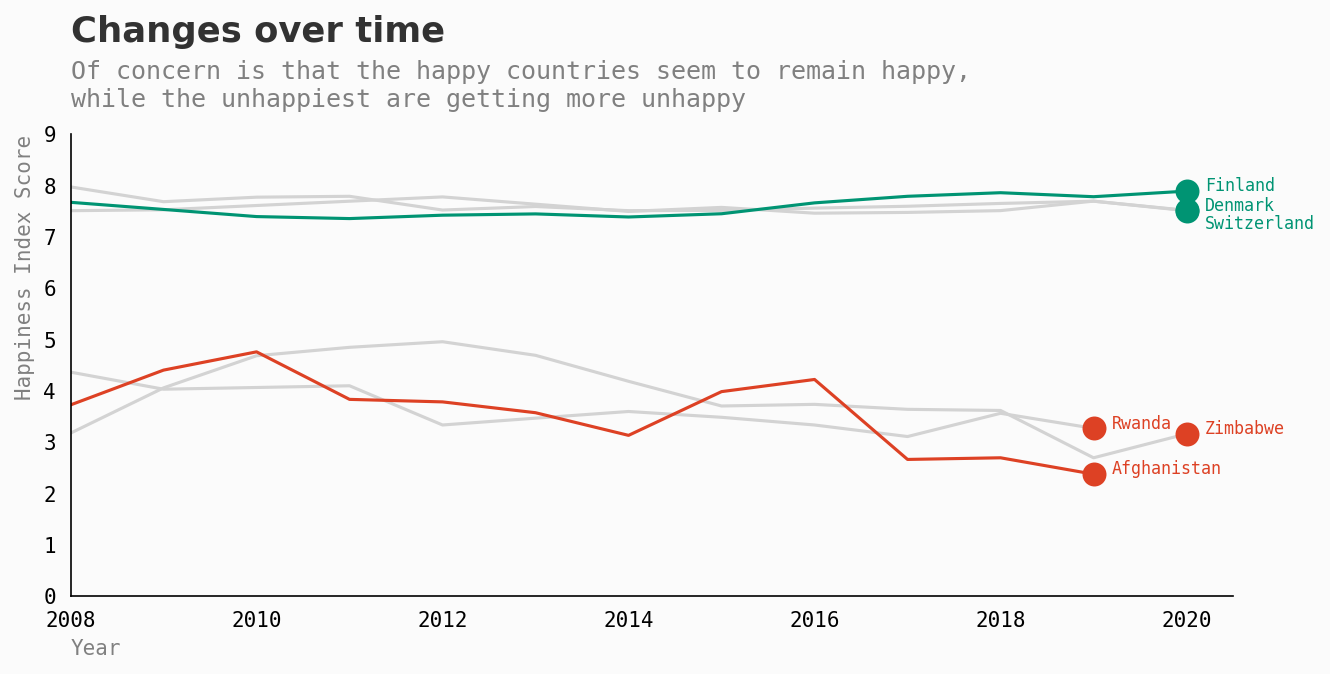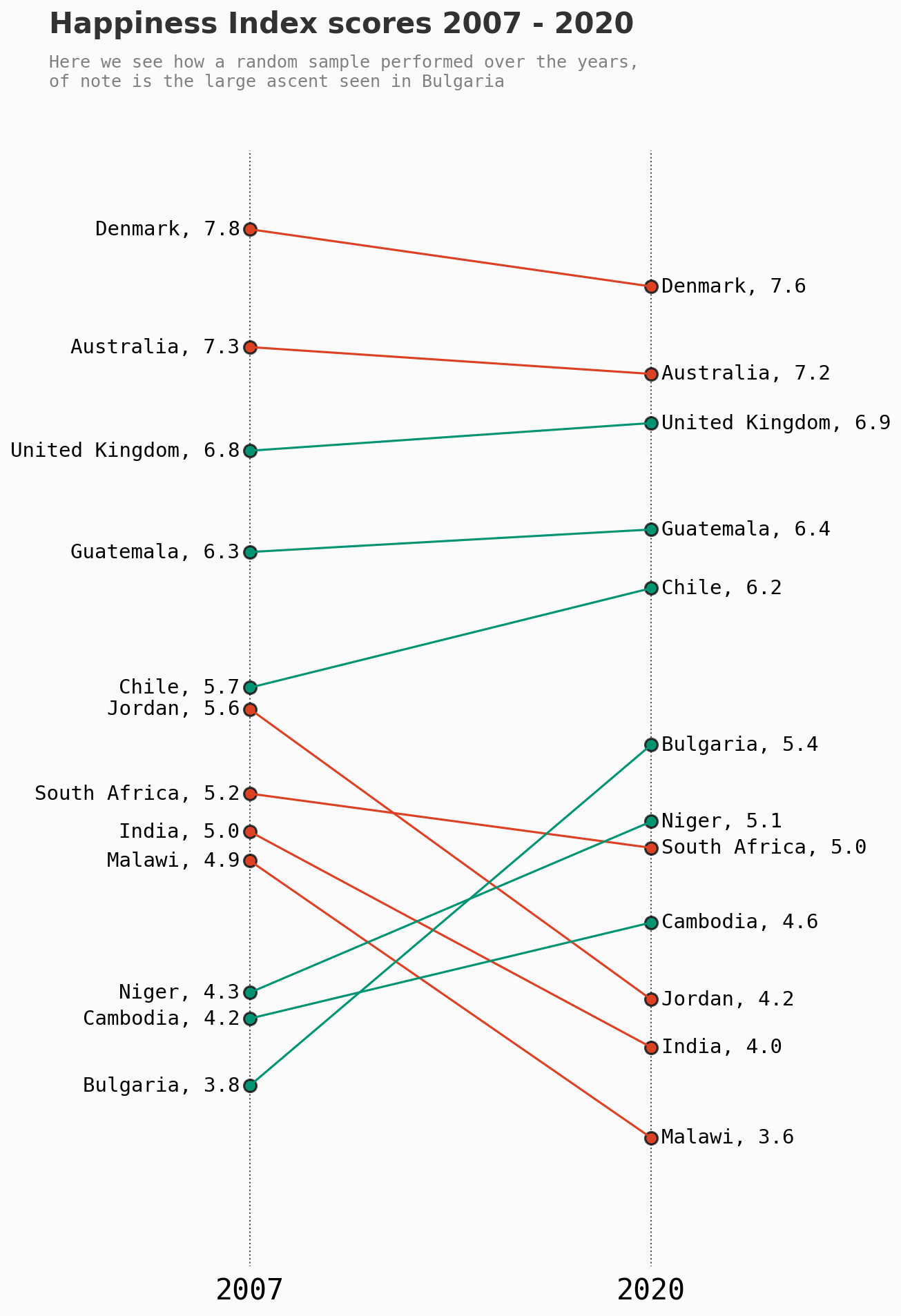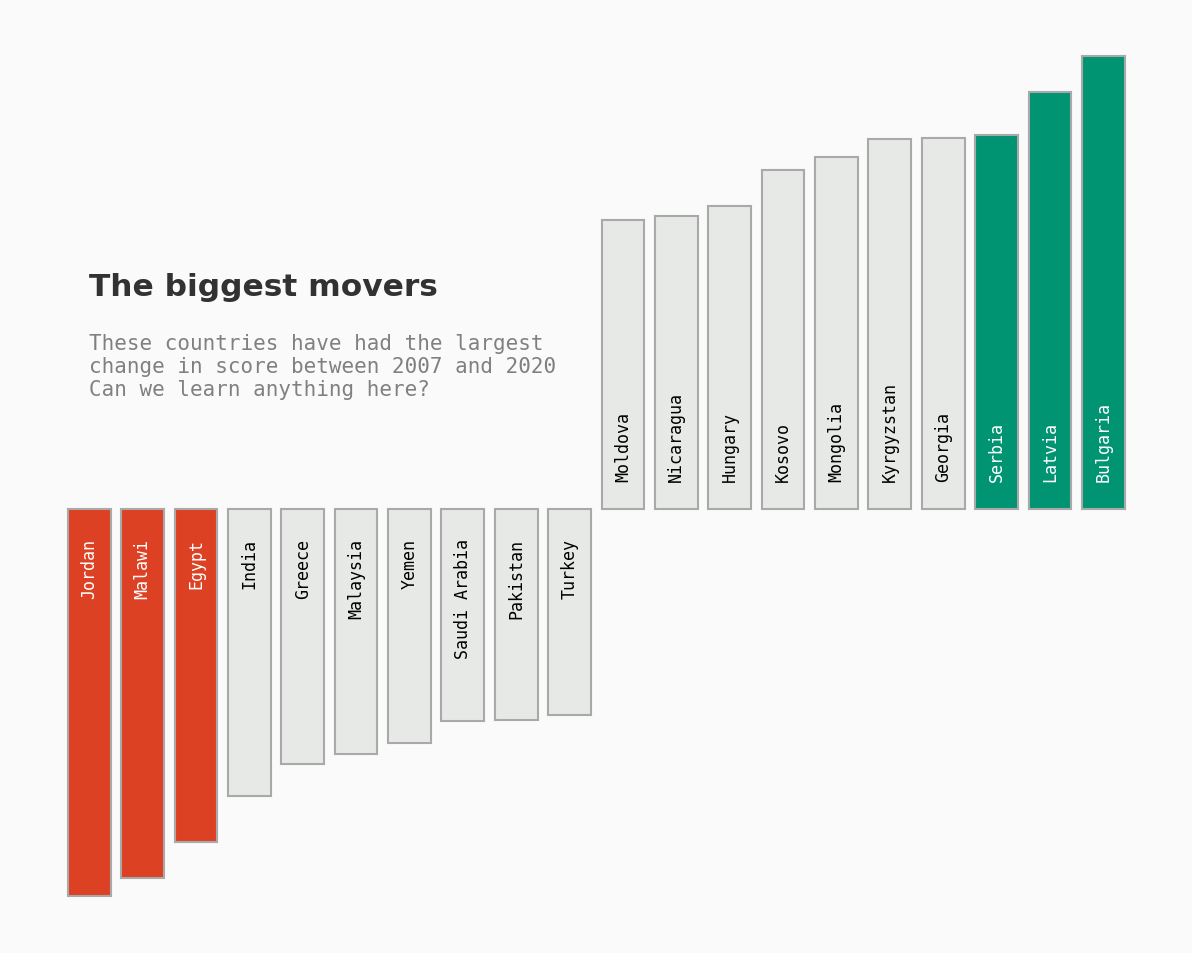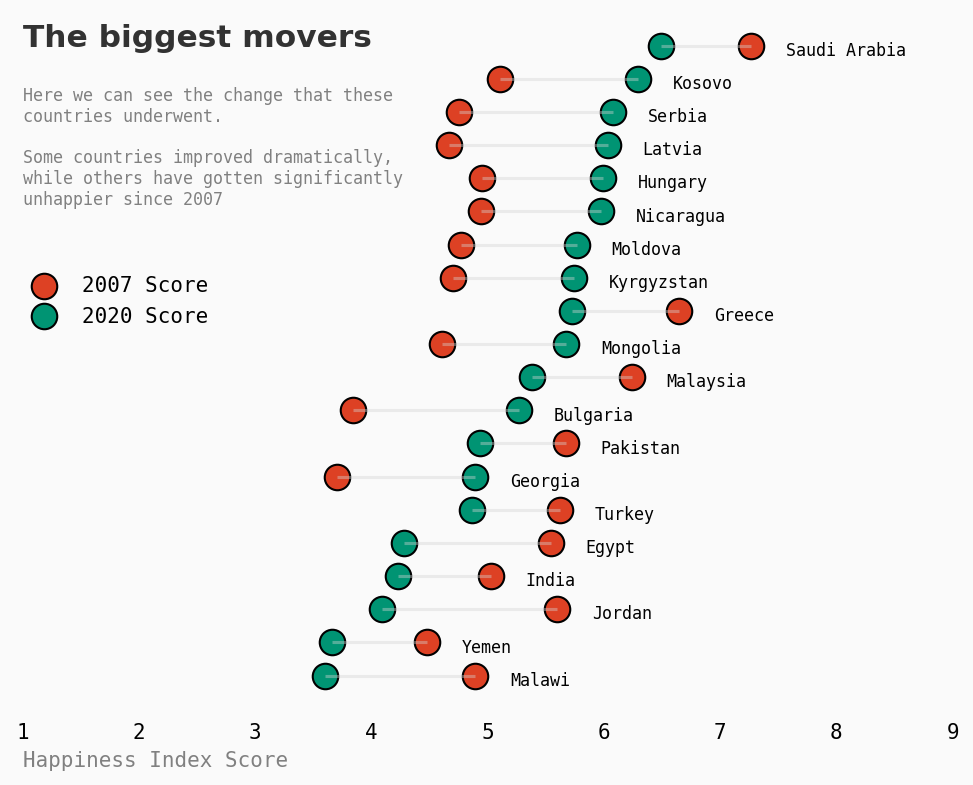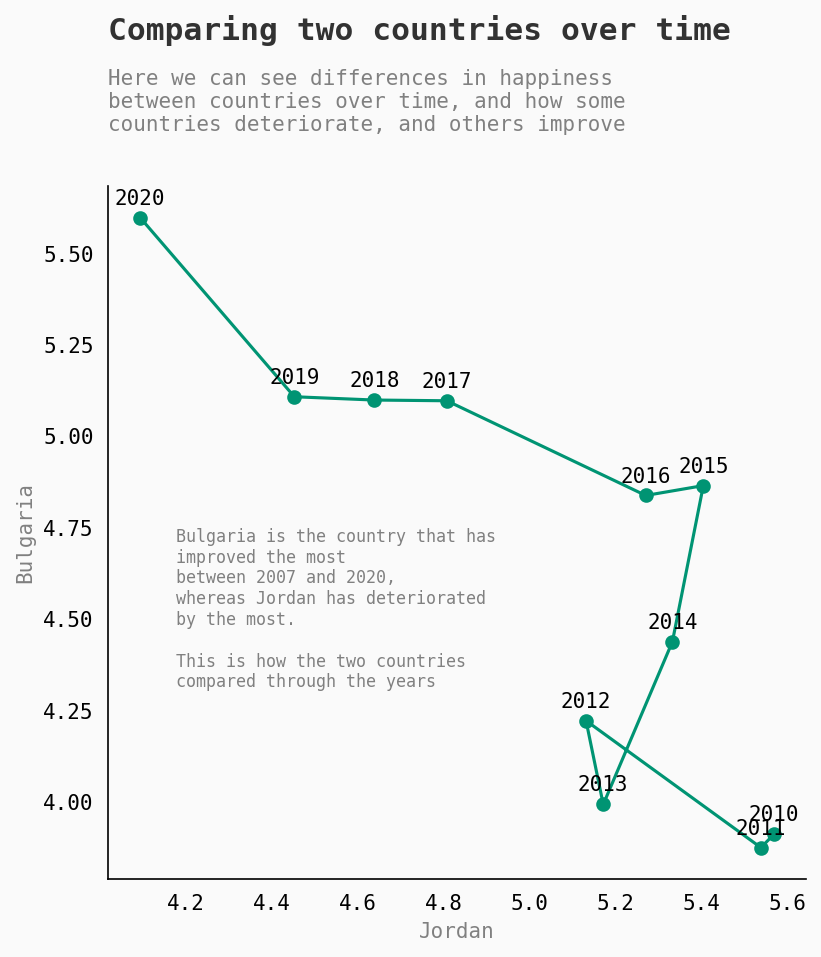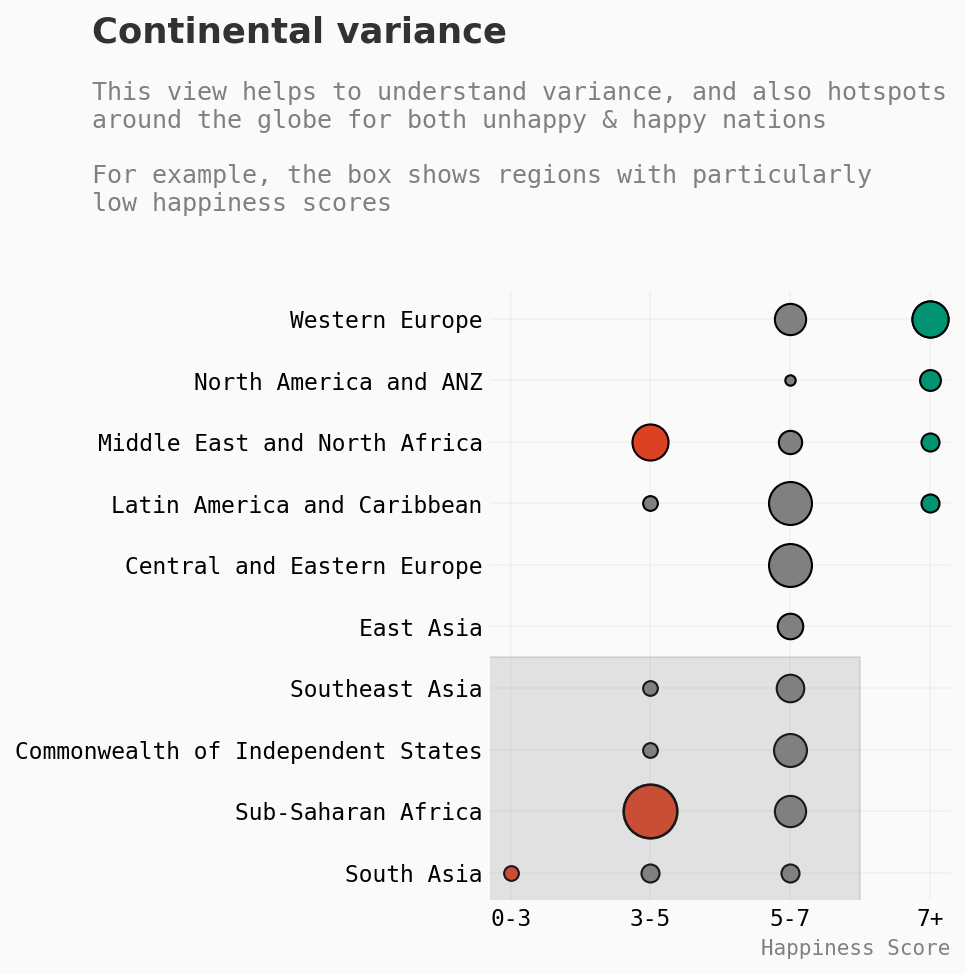作者: JOSH



## 准备工作#

pip3 install pywaffle, geopandas, pycountry


import pandas as pd
import warnings
warnings.filterwarnings("ignore")
import matplotlib
import matplotlib.pyplot as plt

#get data

safety = df.copy()

# 统一不同数据中的字段名renaming columns for easier merge later
df.rename(columns={'Country name': 'Country'}, inplace=True)
df2.rename(columns={'Country name': 'Country'}, inplace=True)
pop.rename(columns={'Country (or dependency)': 'Country'}, inplace=True)

#might use later
temporal = temporal.fillna(0).astype(int)

# colours
low_c = '#dd4124'
high_c = '#009473'
plt.rcParams["font.family"] = "monospace"


## 初始概览#

# inspiration ; https://www.kaggle.com/gaetanlopez/how-to-make-clean-visualizations
# changed code signif.

fig = plt.figure(figsize=(6,3),dpi=150)
gs.update(wspace=0.2, hspace=0.4)

background_color = "#fafafa"
fig.patch.set_facecolor(background_color) # figure background color
ax0.set_facecolor(background_color)

ax0.text(1.167,0.85,"2021 World Happiness Index",color='#323232',fontsize=28, fontweight='bold', fontfamily='sanserif',ha='center')
ax0.text(1.13,-0.35,"stand-out facts",color='lightgray',fontsize=28, fontweight='bold', fontfamily='monospace',ha='center')

ax0.text(0,0.4,"Finland",color=high_c,fontsize=25, fontweight='bold', fontfamily='monospace',ha='center')
ax0.text(0,0.1,"Happiest",color='gray',fontsize=15, fontfamily='monospace',ha='center')

ax0.text(0.77,0.4,"9 of top 10",color=high_c,fontsize=25, fontweight='bold', fontfamily='monospace',ha='center')
ax0.text(0.75,0.1,"in Europe",color='gray',fontsize=15, fontfamily='monospace',ha='center')

ax0.text(1.5,0.4,"7 of bottom 10",color=low_c,fontsize=25, fontweight='bold', fontfamily='monospace',ha='center')
ax0.text(1.5,0.1,"in Africa",color='gray',fontsize=15, fontfamily='monospace',ha='center')

ax0.text(2.25,0.4,"Afghanistan",color=low_c,fontsize=25, fontweight='bold', fontfamily='monospace',ha='center')
ax0.text(2.25,0.1,"Unhappiest",color='gray',fontsize=15, fontfamily='monospace',ha='center')

ax0.set_yticklabels('')
ax0.set_xticklabels('')
ax0.tick_params(axis='both',length=0)

for s in ['top','right','left','bottom']:
ax0.spines[s].set_visible(False)

import matplotlib.lines as lines
l1 = lines.Line2D([0.15, 1.95], [0.67, 0.67], transform=fig.transFigure, figure=fig,color = 'gray', linestyle='-',linewidth = 1.1, alpha = .5)
fig.lines.extend([l1])
l2 = lines.Line2D([0.15, 1.95], [0.07, 0.07], transform=fig.transFigure, figure=fig,color = 'gray', linestyle='-',linewidth = 1.1, alpha = .5)
fig.lines.extend([l2])

plt.show()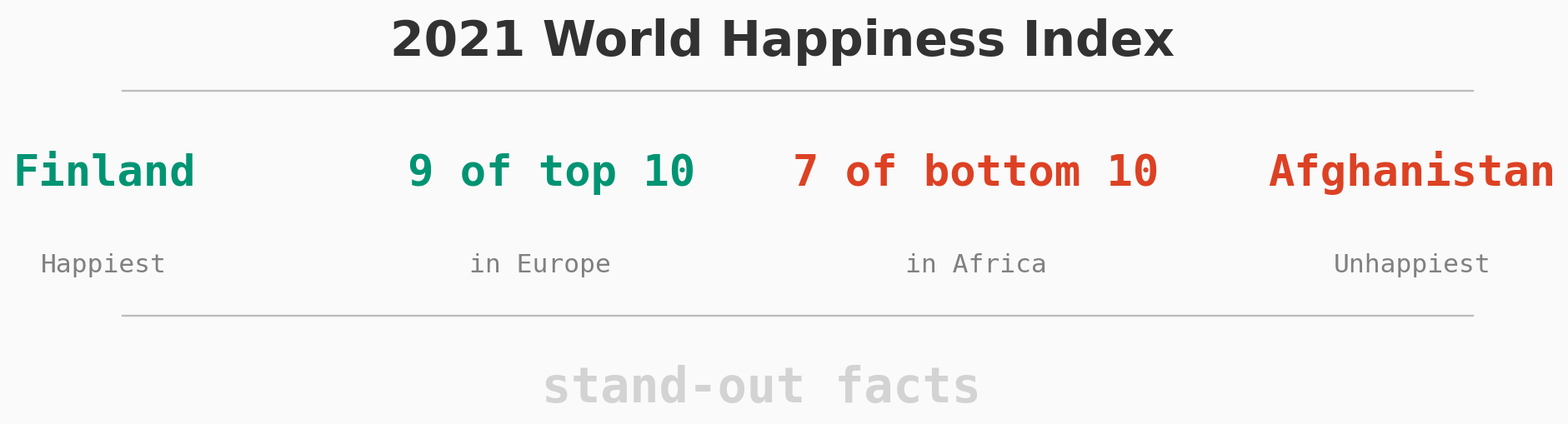## 世界上最幸福的国家是哪些？#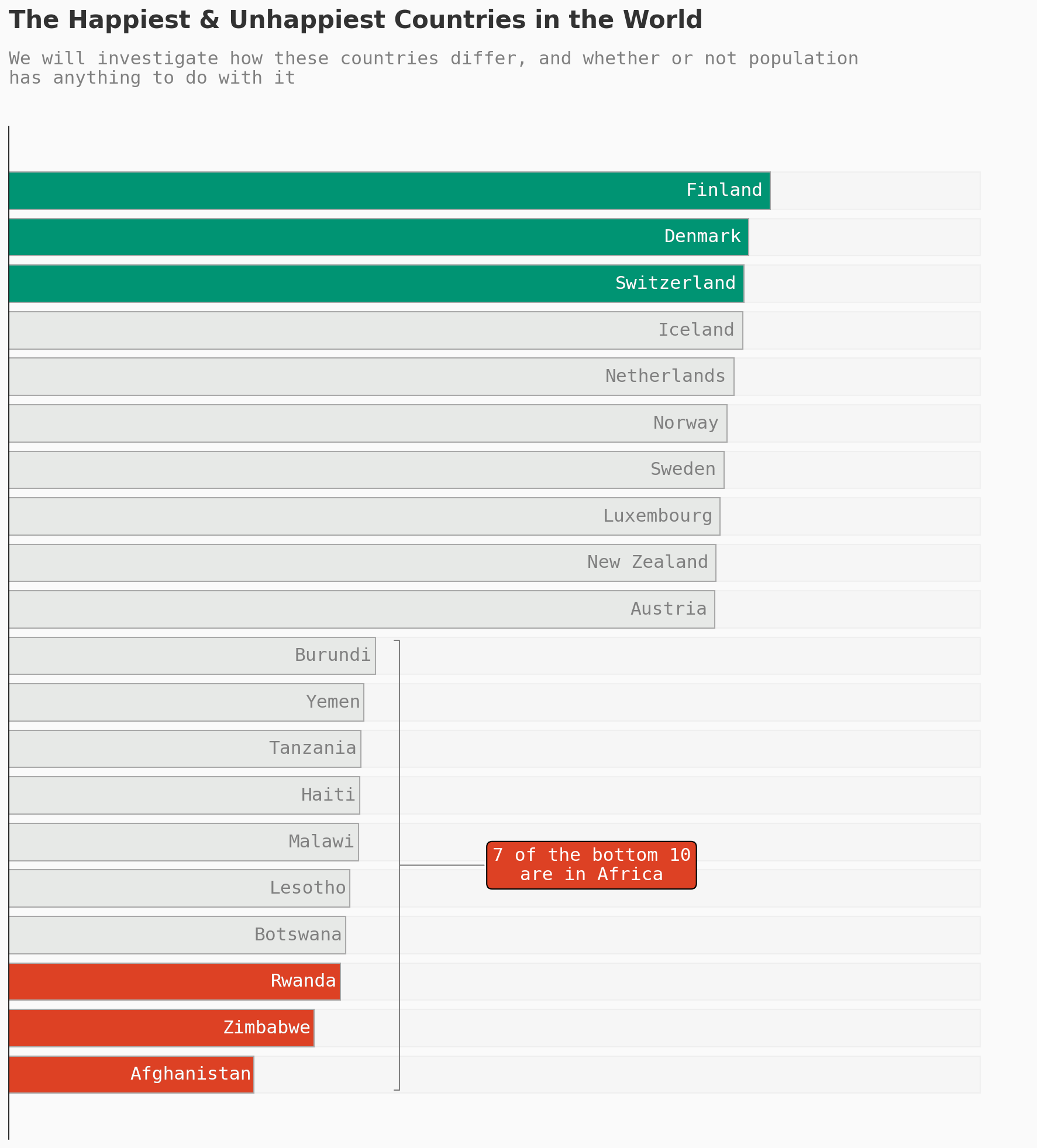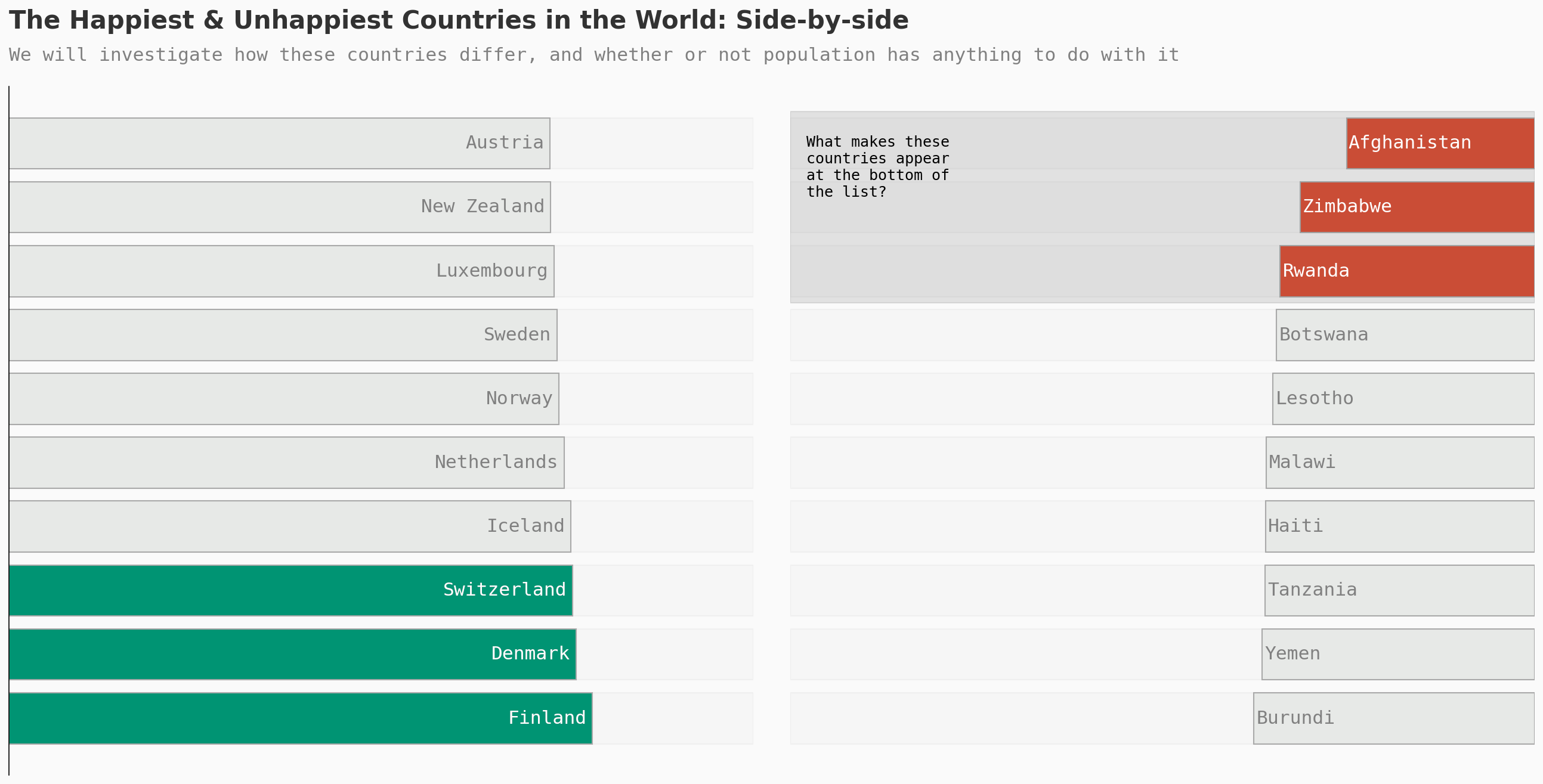happiness_mean = df['Ladder score'].mean()

df['lower_happy'] = df['Ladder score'].apply(lambda x: 0 if x < happiness_mean else 1)


## 这种情况经常发生吗？#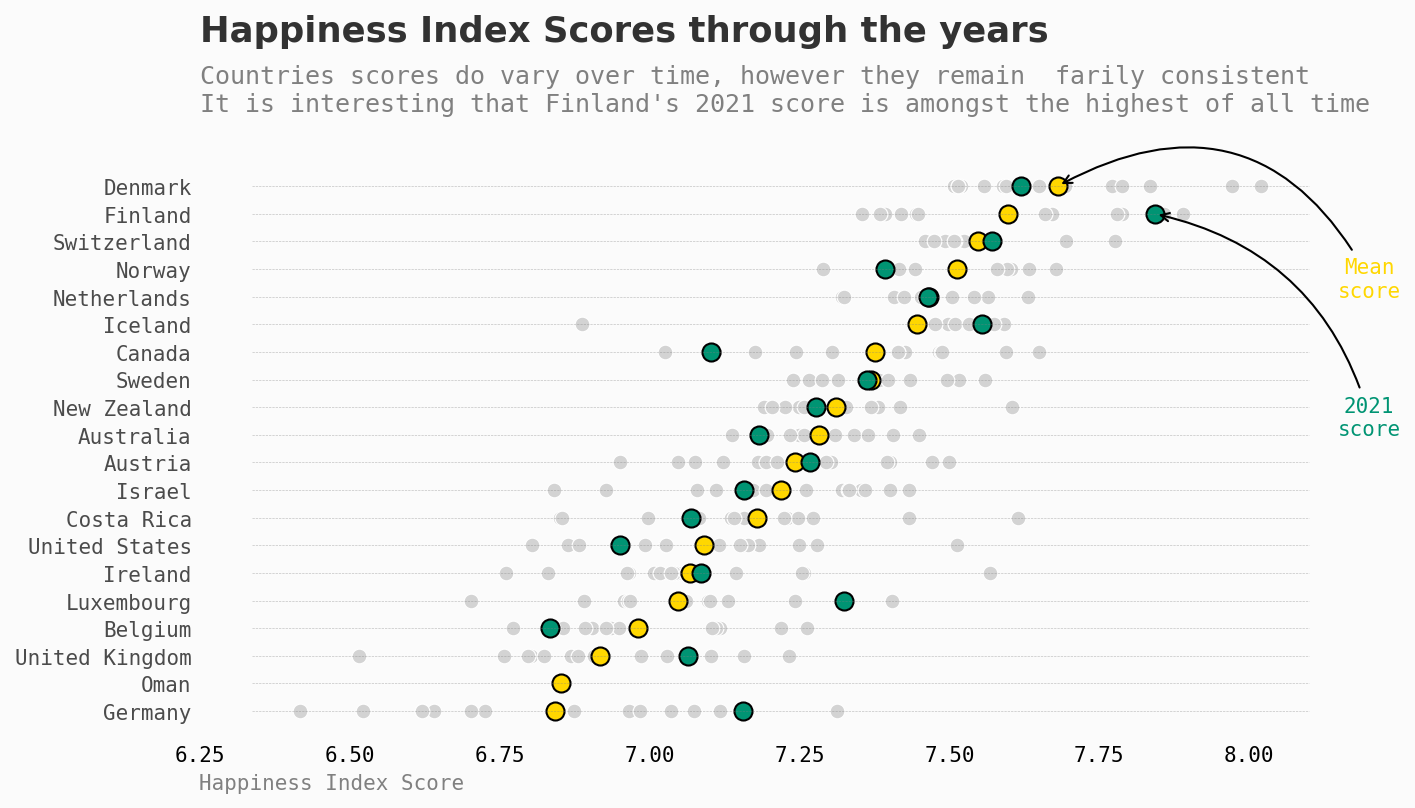## 为什么会有差异？#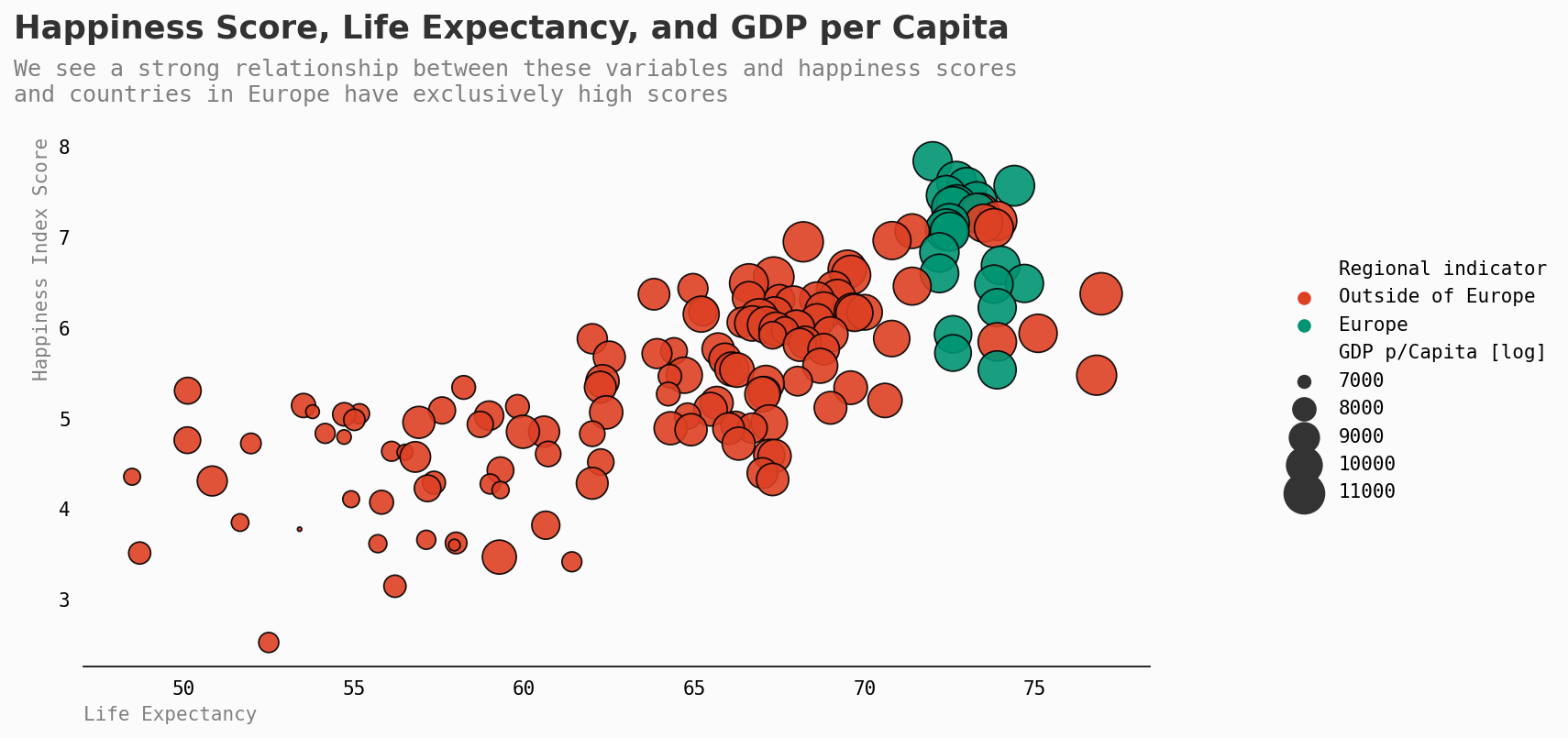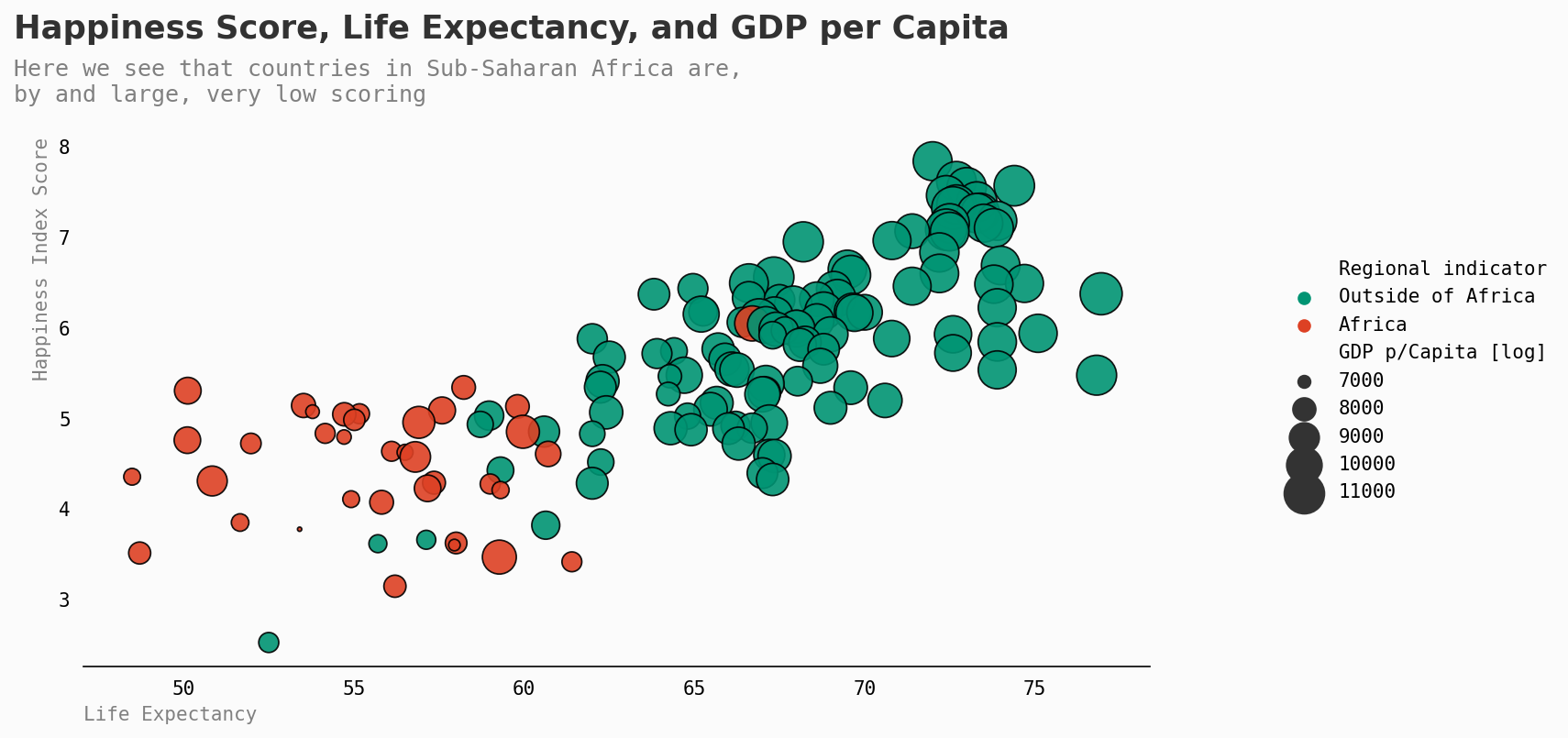## 其他因素#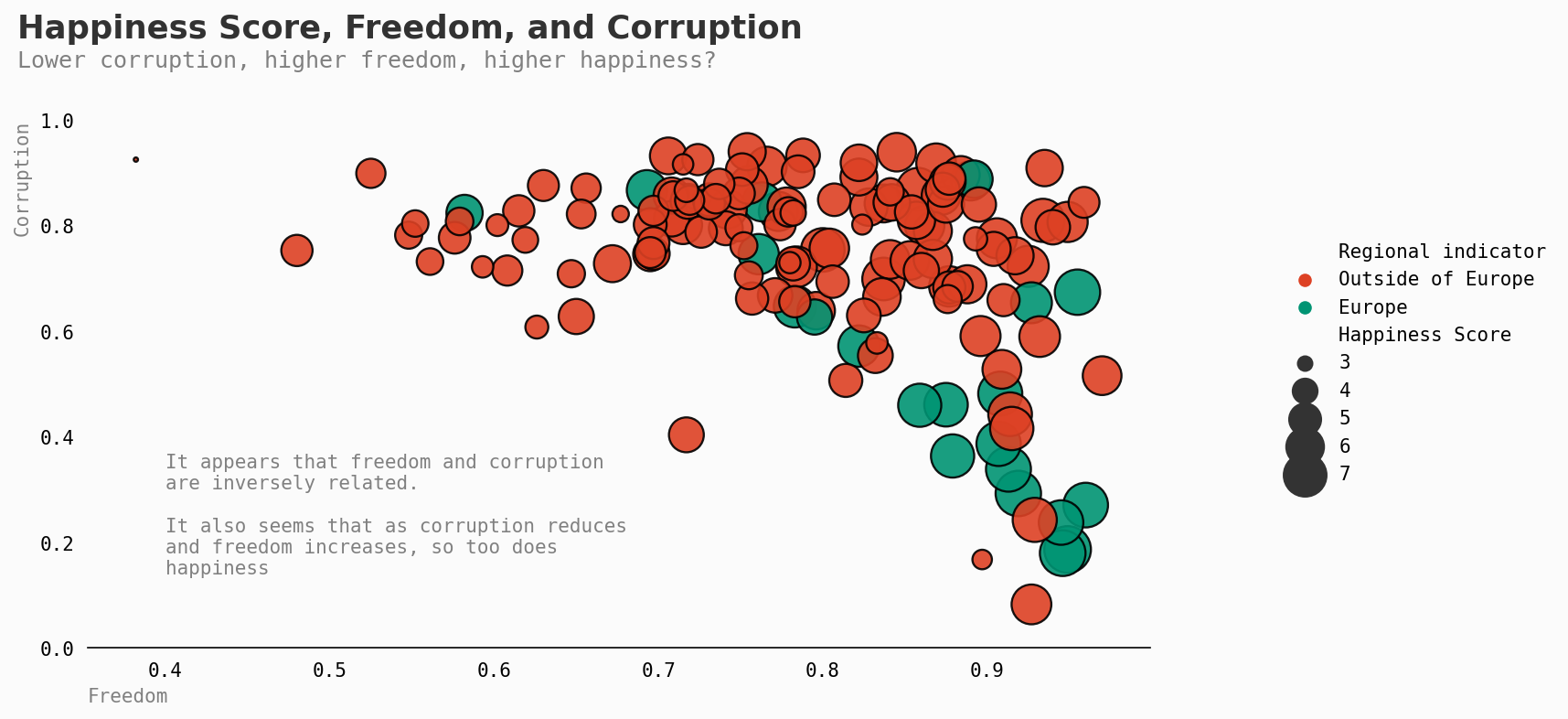## 大陆视角#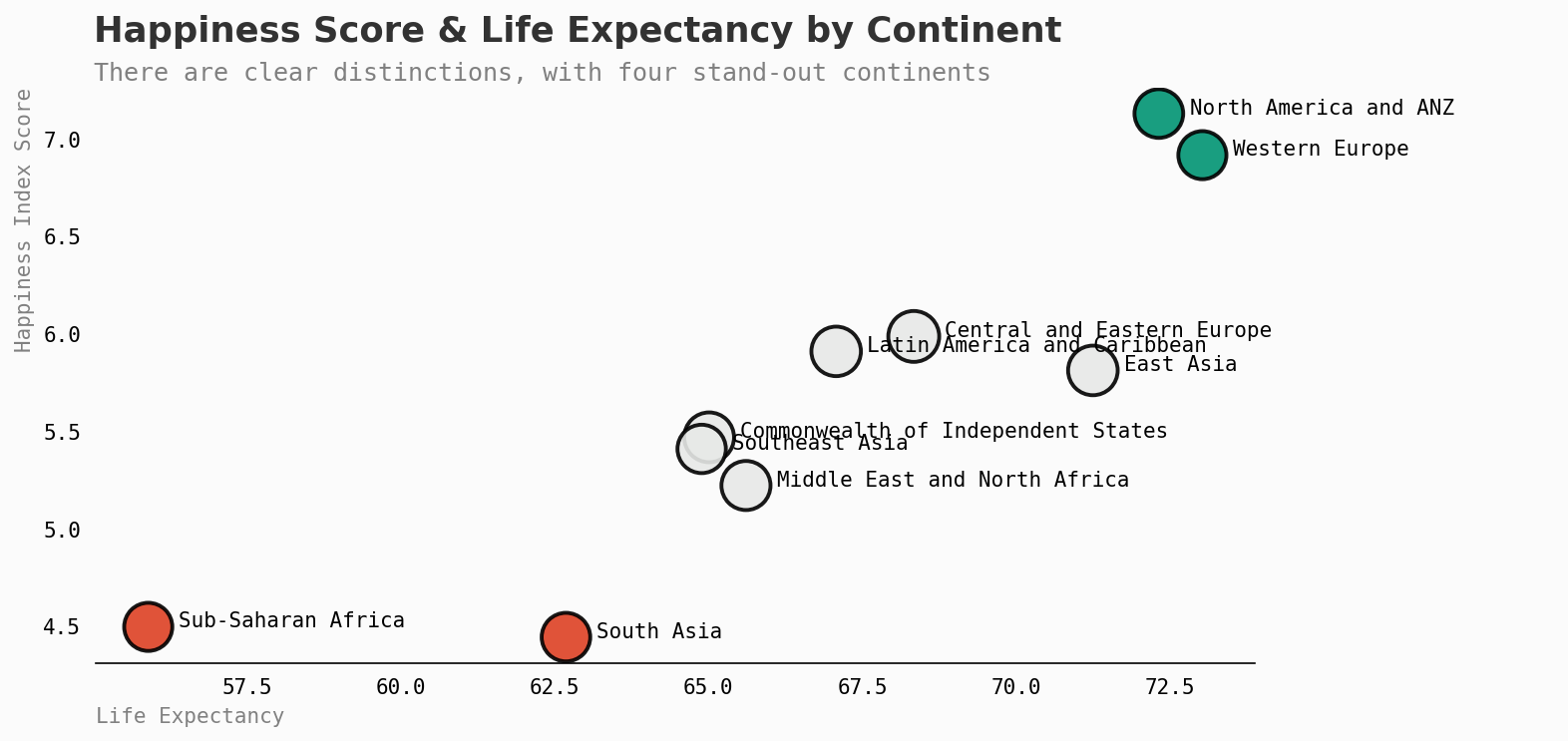continent_score = df.groupby('Regional indicator')[['Healthy life expectancy','Logged GDP per capita','Perceptions of corruption','Freedom to make life choices','Ladder score']].mean().mean(axis=1).sort_values(ascending=True)[:10]

df_bottom = df.groupby('Country')[['Logged GDP per capita','Perceptions of corruption','Freedom to make life choices','Social support','Ladder score']].mean().sort_values(by='Ladder score',ascending=True)[:10]

df_bottom['Logged GDP per capita'] = df_bottom['Logged GDP per capita']/10

categorical = [var for var in df.columns if df[var].dtype=='O']
continuous = [var for var in df.columns if df[var].dtype!='O']

#refined
continuous = ['Logged GDP per capita',
'Social support',
'Healthy life expectancy',
'Freedom to make life choices',
'Generosity',
'Perceptions of corruption']


## 高于和低于平均幸福水平的差异#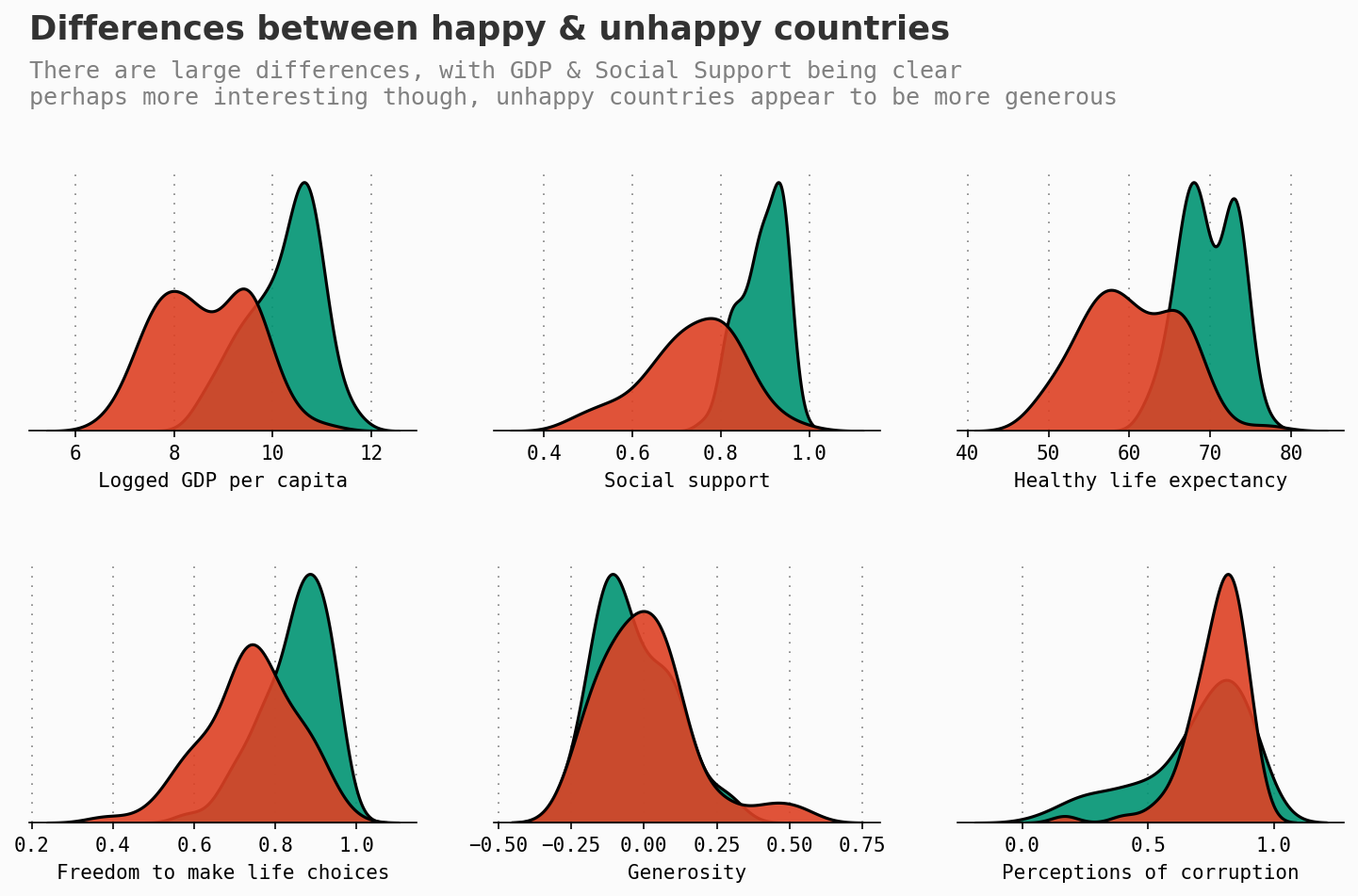## 全球视角#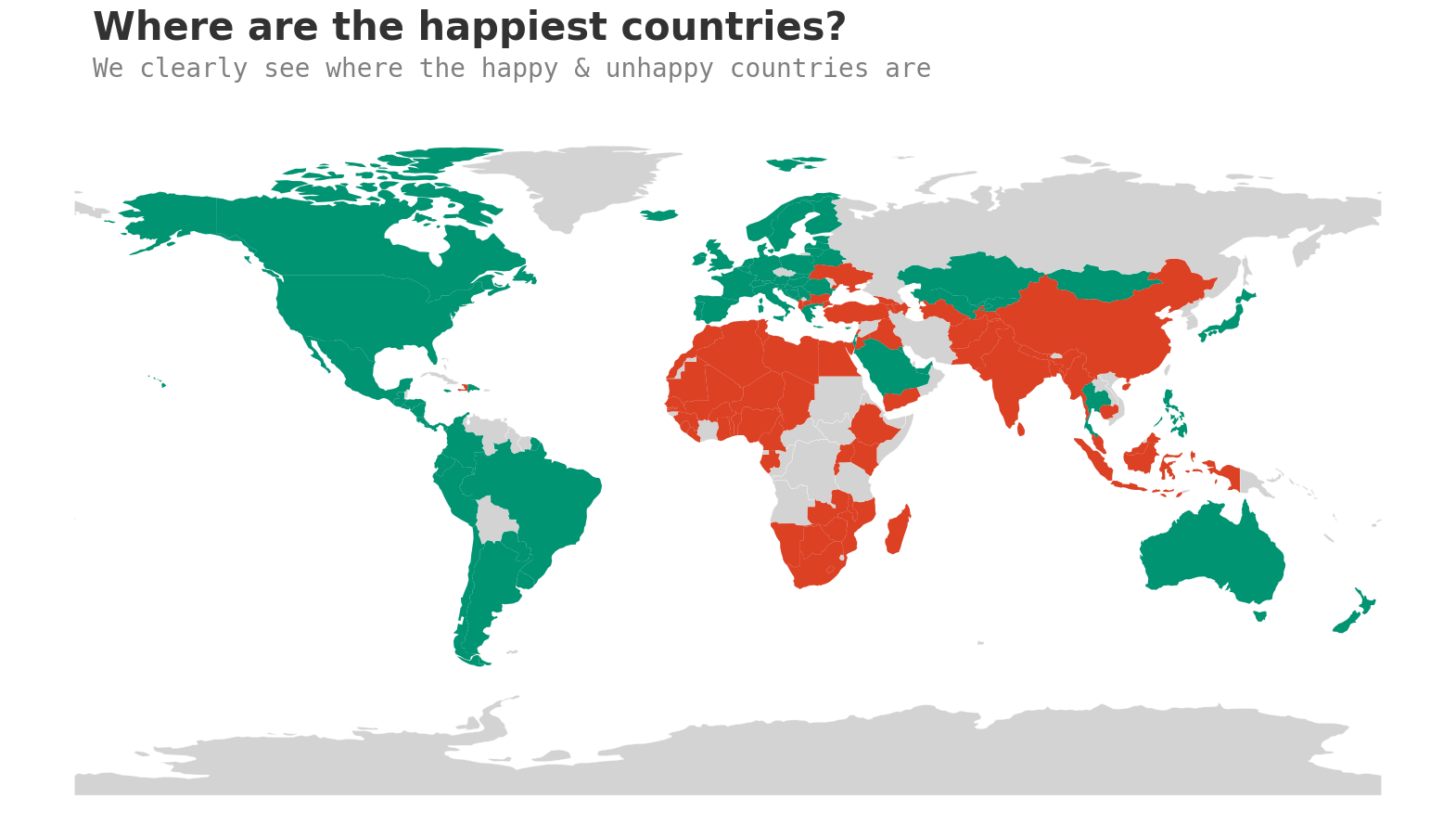## 人口#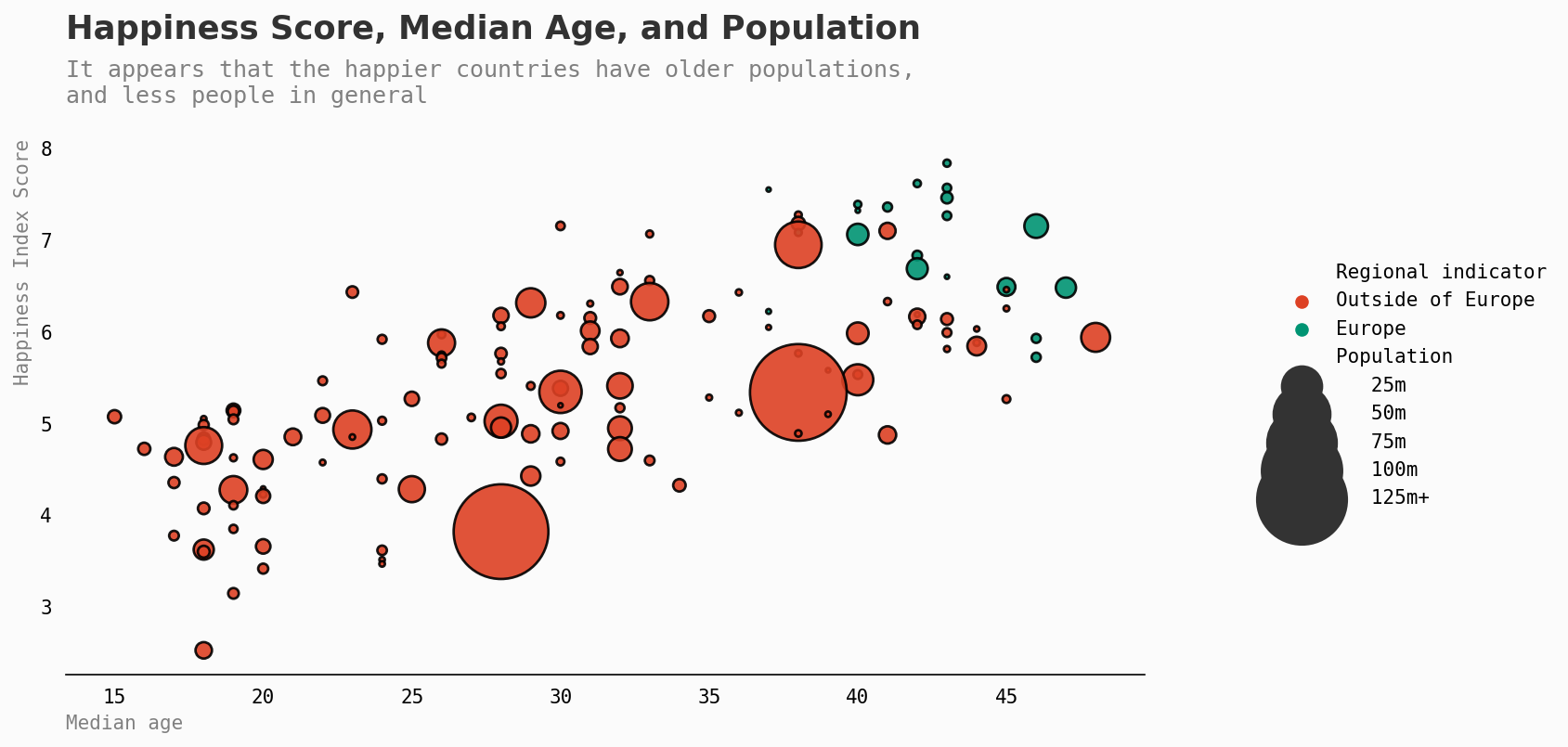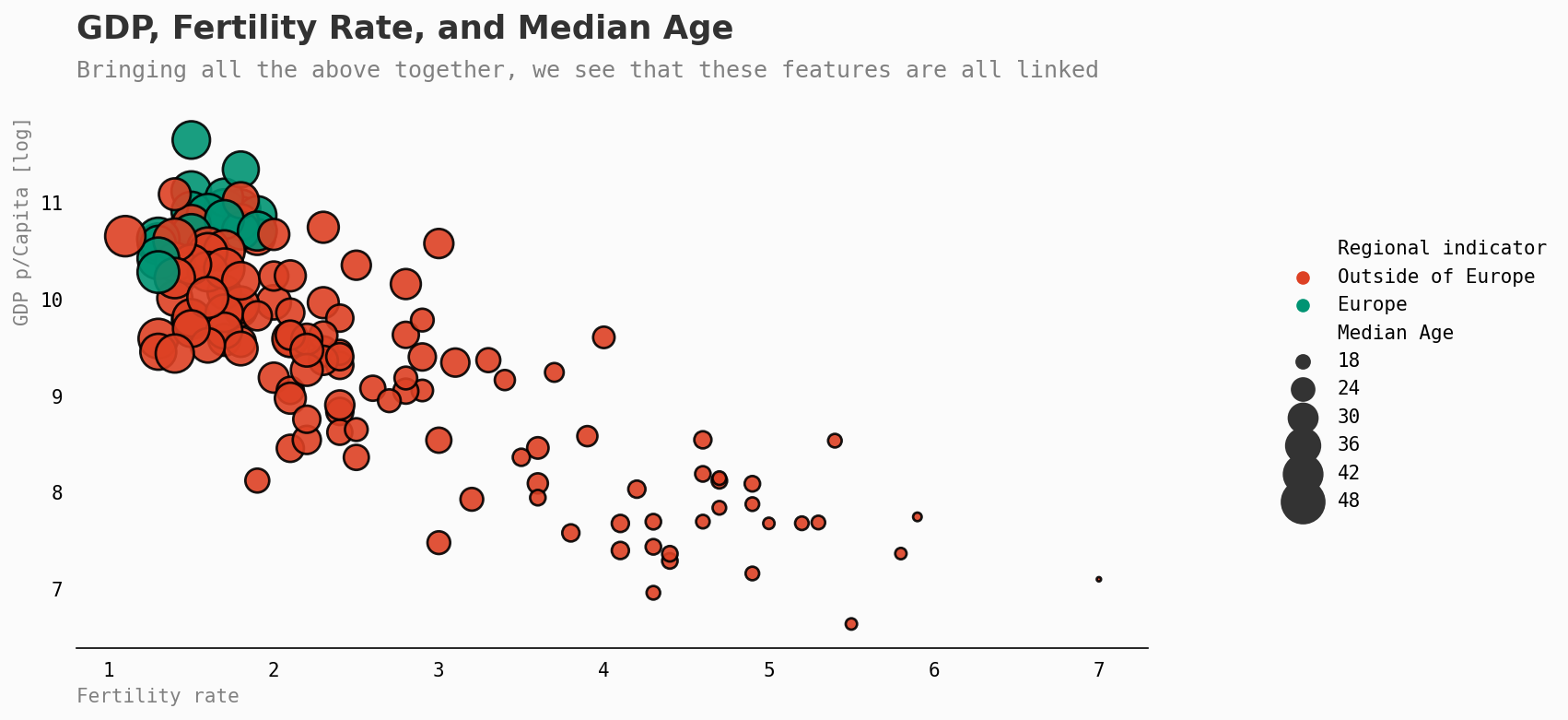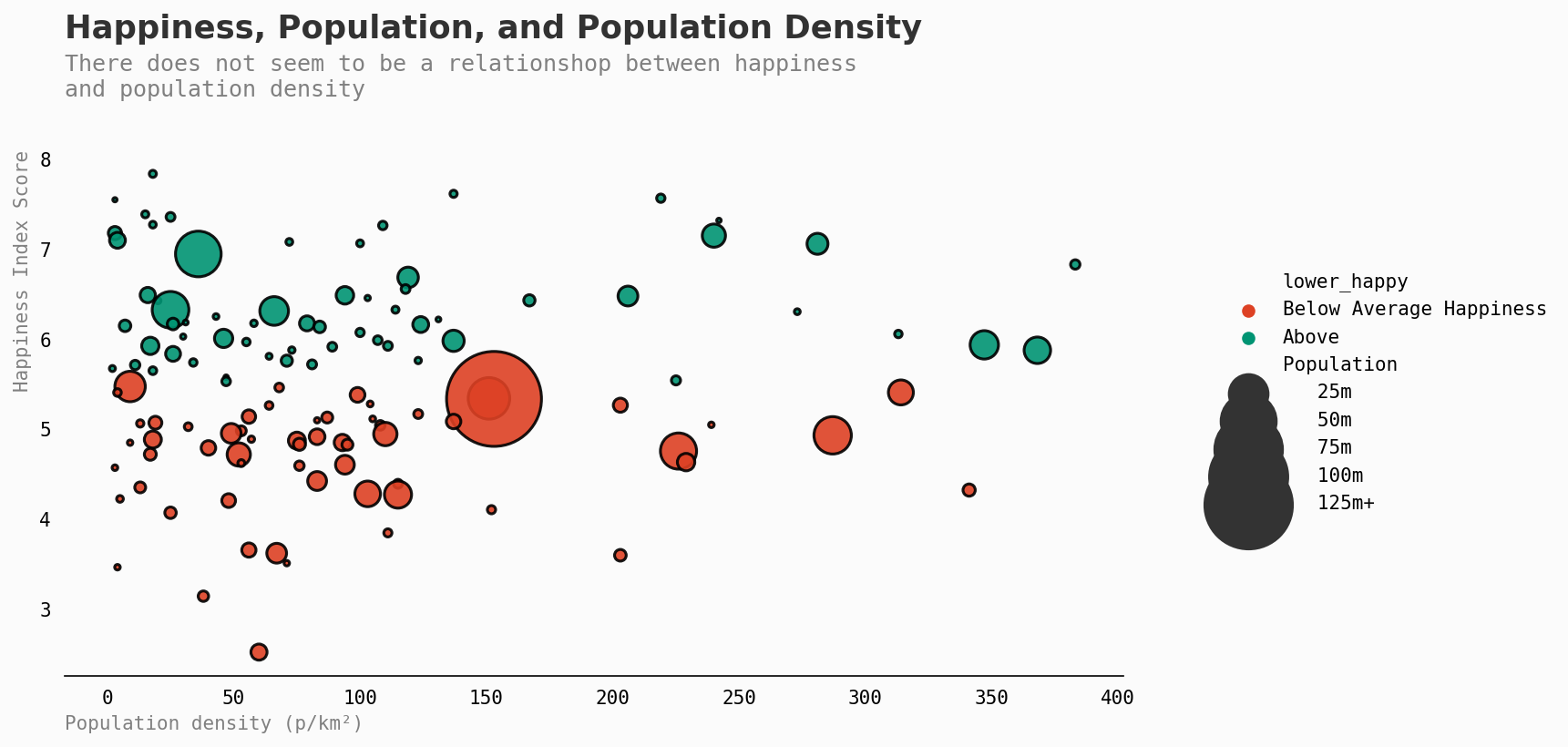## 随着时间的推移，有没有变化？#